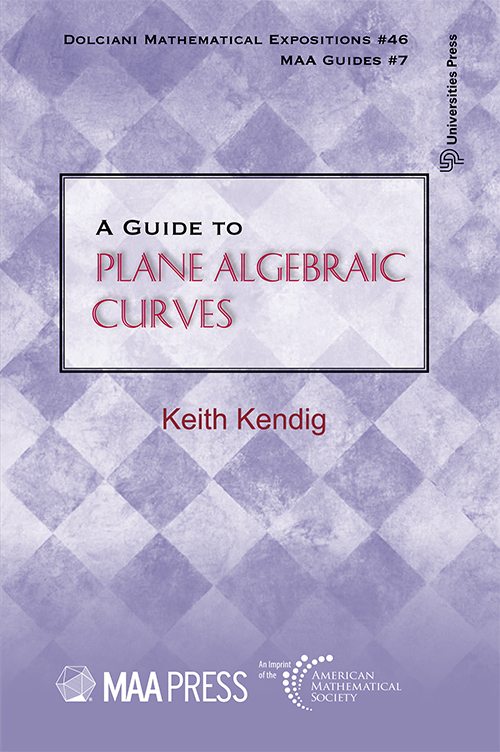## A Guide to Plane Algebraic Curves

#### Price: 700.00

This book is a friendly introduction to plane algebraic curves. It emphasizes geometry and intuition, and the presentation is kept concrete. You'll find an abundance of pictures and examples to help develop your intuition about the subject, which is so basic to understanding and asking fruitful questions. Highlights of the elementary theory are covered, which for some could be an end in itself, and for others an invitation to investigate further. Proofs, when given, are mostly sketched, some in more detail, but typically with less. References to texts that provide further discussion are often included. Computer algebra software has made getting around in algebraic geometry much easier. Algebraic curves and geometry are now being applied to areas such as cryptography, complexity and coding theory, robotics, biological networks, and coupled dynamical systems. Algebraic curves were used in Andrew Wiles' proof of Fermat's Last Theorem, and to understand string theory, you need to know some algebraic geometry. There are other areas on the horizon for which the concepts and tools of algebraic curves and geometry hold tantalizing promise. This introduction to algebraic curves will be appropriate for a wide segment of scientists and engineers wanting an entrance to this burgeoning subject.

#### Contributors (Author(s), Editor(s), Translator(s), Illustrator(s) etc.)

Keith Kendig, Cleveland State University

Preface
1 A Gallery of Algebraic Curves
1.1 Curves of Degree One and Two
1.2 Curves of Degree Three and Higher
1.3 Six Basic Cubics
1.4 Some Curves in Polar Coordinates
1.5 Parametric Curves
1.6 The Resultant
1.7 Back to an Example
1.8 Lissajous Figures
1.9 Morphing Between Curves
1.10 Designer Curves

2 Points at Infinity
2.2 Examples
2.3 A Basic Picture
2.4 Basic Definitions
2.5 Further Examples

3 From Real to Complex
3.1 Definitions
3.2 The Idea of Multiplicity; Examples
3.3 A Reality Check
3.4 A Factorization Theorem for Polynomials in C [x; y]
3.5 Local Parametrizations of a Plane Algebraic Curve
3.6 Definition of Intersection Multiplicity for Two Branches
3.7 An Example
3.8 Multiplicity at an Intersection Point of Two Plane Algebraic Curves
3.9 Intersection MultiplicityWithout Parametrizations
3.10 B´ezout’s theorem
3.11 B´ezout’s theorem Generalizes the Fundamental Theorem of Algebra
3.12 An Application of B´ezout’s theorem: Pascal’s theorem

4 Topology of Algebraic Curves in P2.(C)
4.1 Introduction
4.2 Connectedness
4.3 Algebraic Curves are Connected
4.4 Orientable Two-Manifolds
4.5 Nonsingular Curves are Two-Manifolds
4.6 Algebraic Curves are Orientable
4.7 The Genus Formula

5 Singularities
5.1 Introduction
5.2 Definitions and Examples
5.3 Singularities at Infinity
5.4 Nonsingular Projective Curves
5.5 Singularities and Polynomial Degree
5.6 Singularities and Genus
5.7 A More General Genus Formula
5.8 Non-Ordinary Singularities
5.9 Further Examples
5.10 Singularities versus Doing Math on Curves
5.11 The Function Field of an Irreducible Curve
5.12 Birational Equivalence
5.13 Examples of Birational Equivalence
5.14 Space-Curve Models
5.15 Resolving a Higher-Order Ordinary Singularity
5.16 Examples of Resolving an Ordinary Singularity
5.17 Resolving Several Ordinary Singularities

6 The Big Three: C, K, S
6.1 Function Fields
6.2 Compact Riemann Surfaces
6.3 Projective Plane Curves
6.4 f1, f2, f : Curves and Function Fields
6.5 g1, g2, g: Compact Riemann Surfaces and Curves
6.6 h1, h2, h: Function Fields and Compact Riemann Surfaces
6.7 Genus
6.8 Genus 0
6.9 Genus One
6.10 An Analogy
6.11 Equipotentials and Streamlines
6.12 Differentials Generate Vector Fields
6.13 A Major Difference
6.14 Divisors
6.15 The Riemann-Roch theorem

Bibliography
Index